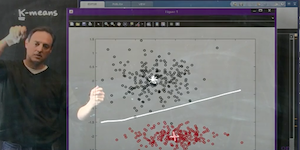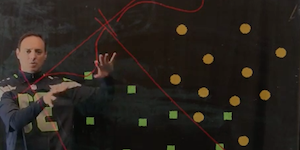# Data Science for Biology

## Clustering and Classification: Basics of Machine Learning

### Lecture 1### [ Part 1 ][ 2 ][ 3 ]

INTRODUCTION TO CLUSTERING AND CLASSIFICATION: This lecture provides an overview of the basic concepts behind supervised and unsupervised learning algorithms. Included is a discussion of k-means and knn (k-nearest neighbors).

MATLAB COMMANDS

`SVD PCA KMEANS KNNSEARCH`

### Lecture 2### [ Part 1 ][ 2 ][ 3 ][ 4 ]

ADVANCED CLUSTERING AND CLASSIFICATION: Classification algorithms such as Gaussian mixture models, Naive Bayes and Boosting are considered in these examples. Training and cross-validation are also considered.

MATLAB COMMANDS

`FITGMDIST CLUSTER FITNAIVEBAYES NB.PREDICT CLASSIFY`

### Lecture 3### [ Part 1 ][ 2 ][ 3 ]

SUPPORT VECTOR MACHINES AND CLASSIFICATION TREES: The classification schemes of support vector machines and regression tress are considered and implemented in MATLAB.

MATLAB COMMANDS

`SVMTRAIN SVMCLASSIFY TREEFIT TREEVAL TREEDISP`